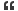A census taker came to a house where a man lived with
The man replied, "The product of their ages is 72, and the
sum of their ages is my house number."
"But that's not enough information," the census taker insisted.
"All right," answered the farmer, "the oldest loves chocolate.Reply With Quote

Hi James,

I am confused with the clue "the oldest loves chocolate". Because all the age group people loves chocolate. Using that clue how can i found that three daughter age? If you give that house number then i can try it..Otherwise i think i can't.

--------------------
sureshReply With Quote

Suresh the problem has sufficient information to solve.

Let me give few hints,

1. "But that's not enough information" the census taker insisted
This is a key statement from census taker

2. The former said "the oldest loves chocolate"
IF you make use of the first hint then you will find that how useful ithis statement is?

Hope this helps uReply With Quote

May be the three daughters age is 3,3,8. If it is not then the answer is 2,6,6.

-----------------
sureshReply With Quote

Excellent Suresh. Please provide ur solution here.Reply With Quote

Actually first i found all the combination of product 72 with three daughter. The combinations are...

(1,2,36) and the sum is 39
(1,3,24) and the sum is 28
(1,4,18) and the sum is 23
(1,6,12) and the sum is 19
(1,8,9) and the sum is 18
(2,3,12) and the sum is 17
(2,4,9) and the sum is 15
(2,6,6) and the sum is 14
(3,3,8) and the sum is 14
(3,4,6) and the sum is 13

In that above table two combination values have the same sum. That is (2,6,6) and (3,3,8)

-------------------
sureshReply With Quote

"sorry i cant understand that why you choose the combination of three daughters be 2,6,6 or 3,3,8 ofcourse their sum is 14 in both the cases so how it could be the solution "

The above question asked by sundarkms in private message....

Here is the answer for his question...

Sundarkms please look at the james hint...

1. "But that's not enough information" the census taker insisted
This is a key statement from census taker

2. The former said "the oldest loves chocolate"
IF you make use of the first hint then you will find that how useful ithis statement is?

Why census taker told that is not enough information? Because he got the same sum for two or more houses. That's why i am taking those two set of values.

I think now sundarkms clear....

--------------------
sureshReply With Quote

the oldest daughter's age is 6
the second one's age is 4
and the third one's age is 3

6 * 4 * 3 = 72 and the sum of the same is 13 which is the house number.Reply With QuoteOriginally Posted by psuresh1982May be the three daughters age is 3,3,8. If it is not then the answer is 2,6,6.

-----------------
sureshOriginally Posted by deepak_pthe oldest daughter's age is 6
the second one's age is 4
and the third one's age is 3

6 * 4 * 3 = 72 and the sum of the same is 13 which is the house number.
Hi,

Hint is in it "the oldest loves chocolate."

the ages are 8,3,3 for sure.

Only oldest loves chocolate means a girl age 8 can eat chocolate. less chances for up-to age 3Reply With Quote

hi, in the information provided by the farmer, he hasn't mentioned that any of his daughters are twins, that gives a hint that the ages should differ from one another. hence, this was the possible solution to go for.
6 + 4 + 3 = 13 and 6 * 4 * 3 = 72.Reply With QuoteOriginally Posted by deepak_phi, in the information provided by the farmer, he hasn't mentioned that any of his daughters are twins, that gives a hint that the ages should differ from one another. hence, this was the possible solution to go for.
6 + 4 + 3 = 13 and 6 * 4 * 3 = 72.
then 9, 8, 1 also correct, am i right?Reply With Quote

We are talking about sum total to be 13 and the product of the total should be 72. if either of the constraint is not there.. then, there are n number of solutions.. and all the above mentioned solutions are correct..Reply With Quote

13.

####Posting Permissions

• You may not post new threads
• You may not post replies
• You may not post attachments
• You may not edit your posts
•